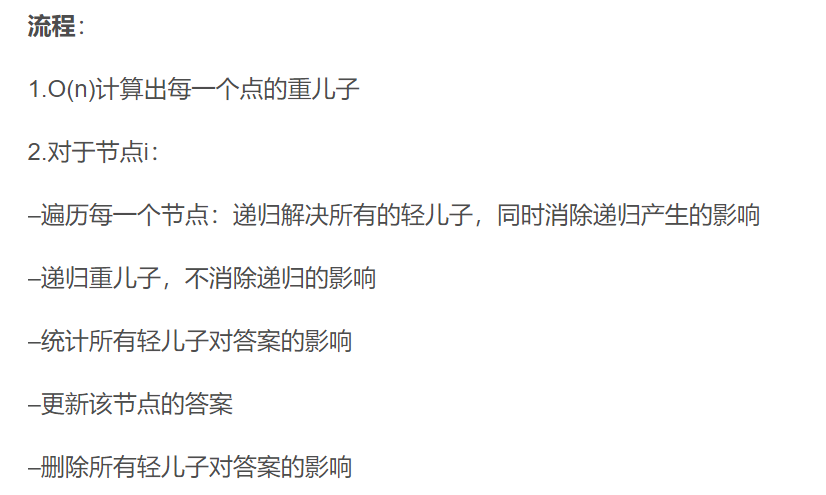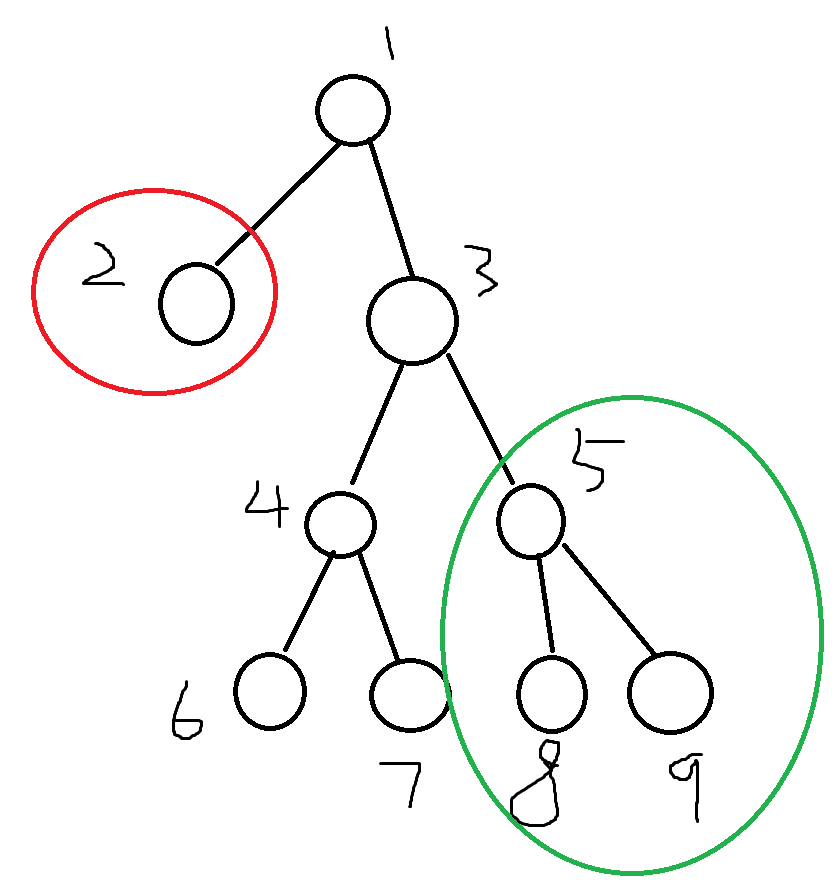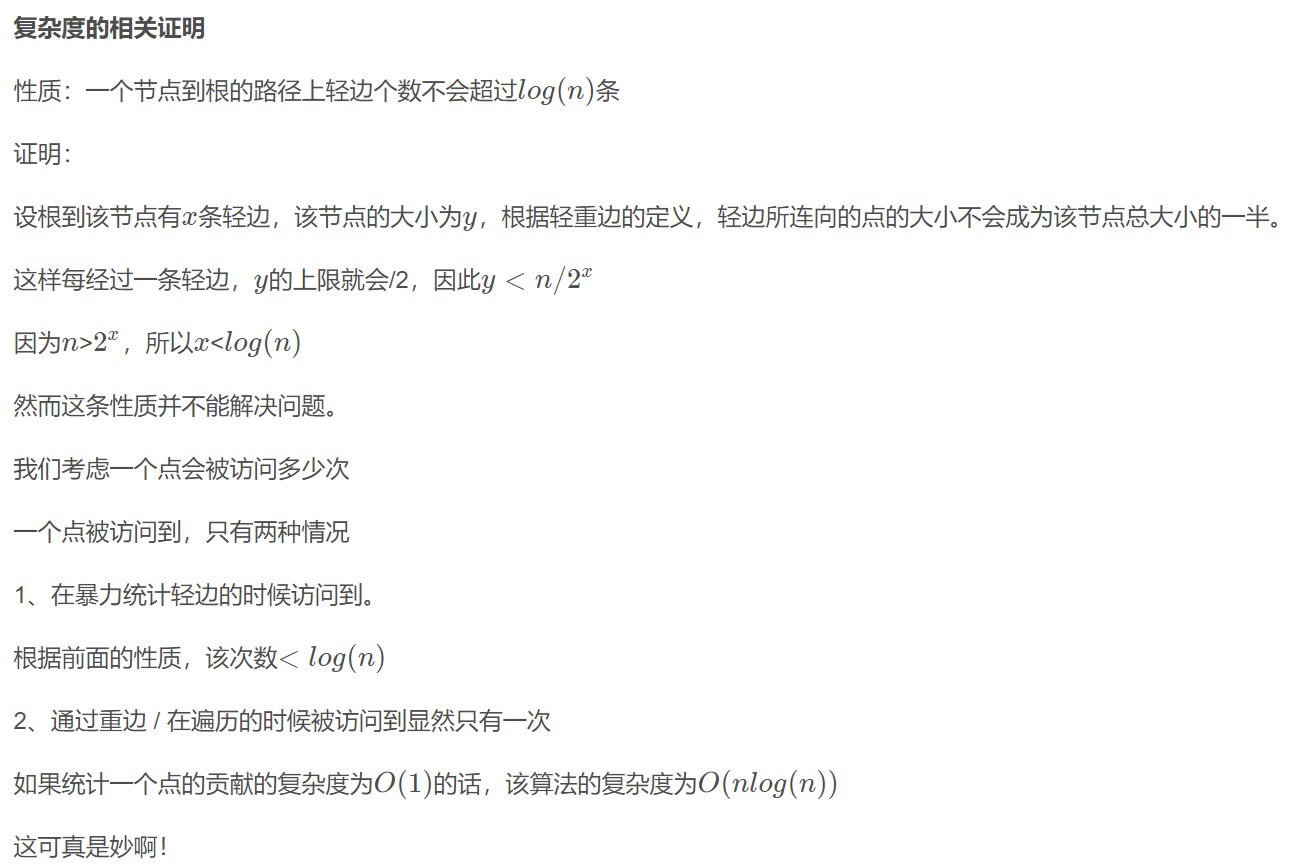# K - Tree 2019icpc南昌K题 （树上启发式合并 dsu on tree）

1.x != y

2.x点不是y点的祖先，y点不是x点的祖先

3.x点和y点的最短距离<=k (看了题解才知道，up to k 原来是小于等于k的意思？？？)

4.设x和y点的公共祖先是z，val【i】表示 i 节点的点权，要求val【z】 * 2 = val【x】 + val【y】PS：提一嘴深度最大值怎么算，假设z是x和y的最近公共祖先，则x和y的距离 = dep【x】 + dep【y】 - 2 * dep【z】，题目要求 x和y的距离<=k, 所以换个位置就是：dep【y】<= k + dep【z】 * 2 - dep【x】代码：

#include<bits/stdc++.h>
#define ll long long
using namespace std;
const int maxn = 1e5 + 7;
int sz[maxn],val[maxn],dep[maxn],son[maxn];
int T[maxn],ls[maxn*200],rs[maxn*200],tr[maxn*200],cnt,n,k;
ll ans;
vector<int>E[maxn];

void dfs1(int u) {//预处理每个节点的大小sz，深度dep和每个节点的重儿子son
sz[u] = 1;
for (auto v:E[u]) {
dep[v] = dep[u] + 1;
dfs1(v);
sz[u] += sz[v];
if(sz[v] > sz[son[u]]) son[u] = v;
}
}
void update(int &rt,int l,int r,int pos,int c) {
if(!rt) rt = ++cnt; // 注意，动态开点
tr[rt] += c;
if(l == r) return ;
int mid = l + r >> 1;
if(pos<=mid) update(ls[rt],l,mid,pos,c);
if(mid<pos) update(rs[rt],mid+1,r,pos,c);
}
ll query(int rt,int l,int r,int L,int R) {
if(!rt) return 0;
if(L<=l && r<=R) return tr[rt];
int mid = l + r >> 1;
ll ans = 0;
if(L<=mid) ans += query(ls[rt],l,mid,L,R);
if(mid<R) ans += query(rs[rt],mid+1,r,L,R);
return ans;
}
void add(int u) {
update(T[val[u]],1,n,dep[u],1);
for (auto v:E[u]) add(v);
}
void del(int u) {//删除u节点及其子树在线段树上的信息
update(T[val[u]],1,n,dep[u],-1);
for (auto v:E[u]) del(v);
}
void gao(int u,int fa) {
int d = k + 2 * dep[fa] - dep[u];//最大深度
int w = 2 * val[fa] - val[u];//另一个点的点权
d = min(d,n);
if(w >= 0 && w <= n) ans += query(T[w],1,n,1,d);
for (auto v:E[u]) gao(v,fa);//子树的每个点都要暴力统计
}
void dfs2(int u) { // 树上启发式合并
for (auto v:E[u]) {  // 1.先跑轻儿子及其子树，跑完后暴力删除
if(v == son[u]) continue;
dfs2(v);//跑轻儿子v及其子树
del(v);//删除轻儿子v及其子树
}
if(son[u]) dfs2(son[u]);//2.跑重儿子，不删
for (auto v:E[u]) {//3.把所有轻儿子都加回来
if(v == son[u]) continue;
gao(v,u);//统计答案 （以u为根节点，其中一个点在v及其 子树）
}
update(T[val[u]],1,n,dep[u],1);//把自己也加上
}

int main() {
int x;
scanf("%d%d",&n,&k);
for (int i=1; i<=n; ++i) {
scanf("%d",&val[i]); //每个点的点权val
}
for (int i=2; i<=n; ++i) {
scanf("%d",&x);
E[x].push_back(i);
}
dep = 1;
dfs1(1);
dfs2(1);//关键看这里
printf("%lld",ans * 2);
return 0;
}
posted @ 2021-10-29 19:33  丿不落良辰  阅读(34)  评论(0编辑  收藏  举报